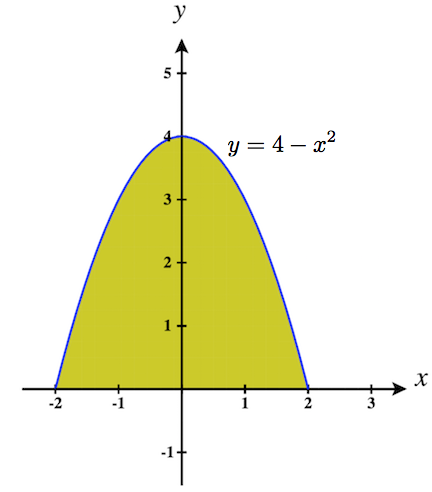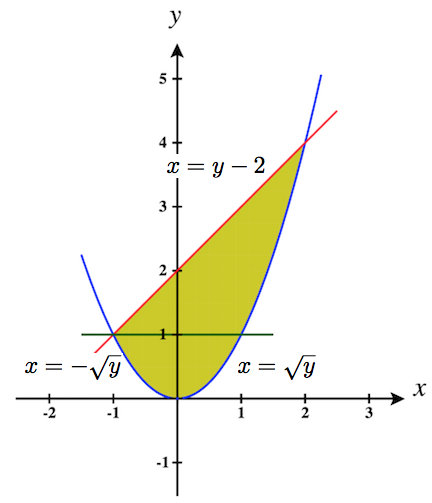$$\newcommand{\id}{\mathrm{id}}$$ $$\newcommand{\Span}{\mathrm{span}}$$ $$\newcommand{\kernel}{\mathrm{null}\,}$$ $$\newcommand{\range}{\mathrm{range}\,}$$ $$\newcommand{\RealPart}{\mathrm{Re}}$$ $$\newcommand{\ImaginaryPart}{\mathrm{Im}}$$ $$\newcommand{\Argument}{\mathrm{Arg}}$$ $$\newcommand{\norm}{\| #1 \|}$$ $$\newcommand{\inner}{\langle #1, #2 \rangle}$$ $$\newcommand{\Span}{\mathrm{span}}$$

# Iterated Integrals and Area (Exercises)

$$\newcommand{\vecs}{\overset { \rightharpoonup} {\mathbf{#1}} }$$ $$\newcommand{\vecd}{\overset{-\!-\!\rightharpoonup}{\vphantom{a}\smash {#1}}}$$$$\newcommand{\id}{\mathrm{id}}$$ $$\newcommand{\Span}{\mathrm{span}}$$ $$\newcommand{\kernel}{\mathrm{null}\,}$$ $$\newcommand{\range}{\mathrm{range}\,}$$ $$\newcommand{\RealPart}{\mathrm{Re}}$$ $$\newcommand{\ImaginaryPart}{\mathrm{Im}}$$ $$\newcommand{\Argument}{\mathrm{Arg}}$$ $$\newcommand{\norm}{\| #1 \|}$$ $$\newcommand{\inner}{\langle #1, #2 \rangle}$$ $$\newcommand{\Span}{\mathrm{span}}$$ $$\newcommand{\id}{\mathrm{id}}$$ $$\newcommand{\Span}{\mathrm{span}}$$ $$\newcommand{\kernel}{\mathrm{null}\,}$$ $$\newcommand{\range}{\mathrm{range}\,}$$ $$\newcommand{\RealPart}{\mathrm{Re}}$$ $$\newcommand{\ImaginaryPart}{\mathrm{Im}}$$ $$\newcommand{\Argument}{\mathrm{Arg}}$$ $$\newcommand{\norm}{\| #1 \|}$$ $$\newcommand{\inner}{\langle #1, #2 \rangle}$$ $$\newcommand{\Span}{\mathrm{span}}$$

## Terms and Concepts

1. When integrating $$f_x(x,y)$$ with respect to $$x$$, the constant of integration C is really which: $$C(x)\text{ or }C(y)$$? What does this mean?

The constant of integration will be $$C(y)$$ since $$y$$ is considered a constant in the integration and thus, the original function $$f$$ could have terms that are functions of $$y$$ that were lost when taking the partial derivative with respect to the variable $$x$$.

2. Integrating an iterated integral is called _________ __________.

iterated integration or multiple integration

3. When evaluating an iterated integral of the form $$\displaystyle \int_a^b \int_{g_1(x)}^{g_2(x)}\,dy\,dx$$, we integrate from _______ to ________, then from _________ to __________.

We integrate from bottom to top, then from left to right. Here, from $$y = g_1(x)$$ to $$y = g_2(x)$$, then from $$x = a$$ to $$x = b$$.
More generally, we integrate from curve to curve and then from point to point.

4. One understanding of an iterated integral is that $$\displaystyle \int_a^b \int_{g_1(x)}^{g_2(x)}\,dy\,dx$$ gives the _______ of a plane region.

area

## Problems

In Exercises 5-10, evaluate the integral and subsequent iterated integral.

5. (a) $$\displaystyle \int_2^5 (6x^2+4xy-3y^2)\,dy$$
(b) $$\displaystyle \int_{-3}^2 \int_2^5 (6x^2+4xy-3y^2)\,dy\,dx$$

(a) $$\displaystyle \int_2^5 (6x^2+4xy-3y^2)\,dy$$ $$= \quad \left(6x^2y + 2xy^2 - y^3\right)\bigg|_2^5$$ $$= \quad 30x^2 + 50x - 125 - (12x^2 + 8x - 8)$$ $$=\quad 18x^2 +42x - 117$$
(b) $$\displaystyle \int_{-3}^2 \int_2^5 (6x^2+4xy-3y^2)\,dy\,dx$$ $$=\quad \displaystyle \int_{-3}^2 \left( 18x^2 +42x - 117 \right)\, dx$$ $$=\quad \left(6x^3 + 21x^2 -117x\right)\bigg|_{-3}^2$$ $$=\quad 48 + 84 - 234 - (-162 +189 +351)$$ $$=\quad -102 - 378$$ $$=\quad 480$$

6. (a) $$\displaystyle \int_0^\pi (2x\cos y +\sin x)\,dx$$
(b) $$\displaystyle \int_{0}^{\pi/2} \int_0^\pi (2x\cos y +\sin x)\,dx\,dy$$

7. (a) $$\displaystyle \int_1^x (x^2y-y+2)\,dy$$
(b) $$\displaystyle \int_0^2 \int_1^x (x^2y-y+2)\,dy\,dx$$

(a) $$\displaystyle \int_1^x (x^2y-y+2)\,dy$$ $$= \quad \left(\frac{x^2y^2}{2} - \frac{y^2}{2} + 2y\right)\bigg|_1^x$$ $$= \quad \frac{x^4}{2} - \frac{x^2}{2} + 2x - (\frac{x^2}{2} - \frac{1}{2} + 2)$$ $$=\quad \frac{x^4}{2} - x^2 + 2x - \frac{3}{2}$$
(b) $$\displaystyle \int_0^2 \int_1^x (x^2y-y+2)\,dy\,dx$$ $$=\quad \displaystyle \int_{0}^2 \left( \frac{x^4}{2} - x^2 + 2x - \frac{3}{2} \right)\, dx$$ $$=\quad \left(\frac{x^5}{10} - \frac{x^3}{3} + x^2 - \frac{3}{2}x\right)\bigg|_{0}^2$$ $$=\quad \frac{32}{10} - \frac{8}{3} + 4 - 3 - 0$$ $$=\quad \frac{96}{30} - \frac{80}{30} + \frac{30}{30}$$ $$=\quad \dfrac{23}{15}$$

8. (a) $$\displaystyle \int_y^{y^2} (x-y)\,dx$$
(b) $$\displaystyle \int_{-1}^1 \int_y^{y^2} (x-y)\,dx\,dy$$

9. (a) $$\displaystyle \int_0^{y} (\cos x \sin y)\,dx$$
(b) $$\displaystyle \int_0^{\pi} \int_0^{y} (\cos x \sin y)\,dx\,dy$$

(a) $$\displaystyle \int_0^{y} (\cos x \sin y)\,dx\quad$$ $$= \quad \left(\sin x \sin y \right)\bigg|_0^y$$ $$= \quad \sin^2 y$$
(b) $$\displaystyle \int_0^{\pi} \int_0^{y} (\cos x \sin y)\,dx\,dy\quad$$ $$=\quad \displaystyle \int_{0}^{\pi} \sin^2 y \, dy\quad$$ $$=\quad \displaystyle \int_{0}^{\pi} \frac{1-\cos 2y}{2}\, dy\quad$$ $$=\quad \dfrac{y - \frac{1}{2}\sin 2y}{2}\bigg|_{0}^{\pi}\quad$$ $$=\quad \frac{\pi}{2} - 0\quad$$ $$=\quad \dfrac{\pi}{2}$$

10. (a) $$\displaystyle \int_0^{x} \left (\frac{1}{1+x^2}\right )\,dy$$
(b) $$\displaystyle \int_1^2 \int_0^{x} \left (\frac{1}{1+x^2}\right )\,dy\,dx$$

In Exercises 11-16, a graph of a planar region $$R$$ is given. Give the iterated integrals, with both orders of integration $$dy\,dx$$ and $$dx\,dy$$, that give the area of $$R$$. Evaluate one of the iterated integrals to find the area.

11.$$\text{Area} = \displaystyle \int_1^4 \int_{-2}^1 1\,dy\,dx\quad$$ $$=\quad \displaystyle \int_{-2}^1 \int_1^4 1\,dx\,dy$$
$$\displaystyle \int_{-2}^1 \int_1^4 1\,dx\,dy\quad$$ $$=\quad \displaystyle \int_{-2}^1 x\bigg|_1^4 \,dy\quad$$ $$=\quad \displaystyle \int_{-2}^1 3 \,dy\quad$$ $$=\quad 3y\bigg|_{-2}^1 \quad$$ $$=\quad 3(3) \quad$$ $$=\quad 9 \,\text{units}^2$$

12.13.$$\text{Area} = \displaystyle \int_2^4 \int_{x-1}^{7-x} 1\,dy\,dx\quad$$ $$=\quad \displaystyle \int_{1}^3 \int_{2}^{y+1} 1\,dx\,dy\quad + \quad \displaystyle \int_{3}^5 \int_{2}^{7-y} 1\,dx\,dy\quad$$
$$\displaystyle \int_2^4 \int_{x-1}^{7-x} 1\,dy\,dx\quad$$ $$=\quad\displaystyle \int_2^4 y\bigg|_{x-1}^{7-x}\,dx\quad$$ $$=\quad\displaystyle\int_2^4 (8 - 2x)\,dx\quad$$ $$=\quad(8x - x^2)\bigg|_2^4 = 32 - 16 - 16 + 4 \quad$$ $$=\quad 4 \,\text{units}^2$$

14.15.$$\text{Area} = \displaystyle \int_0^1 \int_{x^4}^{\sqrt{x}} 1\,dy\,dx\quad$$ $$=\quad \displaystyle \int_{0}^1 \int_{y^2}^{\sqrt{y}} 1\,dx\,dy\quad$$
$$\displaystyle \int_0^1 \int_{x^4}^{\sqrt{x}} \,dy\,dx\quad$$ $$=\quad\displaystyle \int_0^1 y\bigg|_{x^4}^{\sqrt{x}}\,dx\quad$$ $$=\quad\displaystyle\int_0^1 (\sqrt{x} - x^4)\,dx\quad$$ $$=\quad(\frac{2}{3}x^{3/2} - \frac{x^5}{5})\bigg|_0^1 \quad$$ $$=\quad \frac{2}{3} - \frac{1}{5} \quad$$ $$=\quad \frac{10}{15} - \frac{3}{15}$$ $$=\quad \dfrac{7}{15} \,\text{units}^2$$

16.In Exercises 17-22, iterated integrals are given that compute the area of a region R in the $$xy$$-plane. Sketch the region R, and give the iterated integral(s) that give the area of R with the opposite order of integration.

17. $$\displaystyle \int_{-2}^2 \int_0^{4-x^2}\,dy\,dx$$

$$\displaystyle \int_{-2}^2 \int_0^{4-x^2}\,dy\,dx\quad$$ $$=\quad \displaystyle \int_{0}^4 \int_{-\sqrt{4-y}}^{\sqrt{4-y}}\,dx\,dy$$18. $$\displaystyle \int_{0}^1 \int_{5-5x}^{5-5x^2}\,dy\,dx$$

19. $$\displaystyle \int_{-2}^2 \int_0^{2\sqrt{4-y^2}}\,dx\,dy$$

$$\displaystyle \int_{-2}^2 \int_0^{2\sqrt{4-y^2}}\,dx\,dy\quad$$ $$=\quad \displaystyle \int_{0}^4 \int_{-\frac{1}{2}\sqrt{16-x^2}}^{\frac{1}{2}\sqrt{16-x^2}}\,dy\,dx$$20. $$\displaystyle \int_{-3}^3 \int_{-\sqrt{9-x^2}}^{\sqrt{9-x^2}}\,dy\,dx$$
21. $$\displaystyle \int_{0}^1 \int_{-\sqrt{y}}^{\sqrt{y}}\,dx\,dy +\int_1^4 \int_{y-2}^{\sqrt{y}}\,dx\,dy$$
$$\displaystyle \int_{0}^1 \int_{-\sqrt{y}}^{\sqrt{y}}\,dx\,dy +\int_1^4 \int_{y-2}^{\sqrt{y}}\,dx\,dy \quad$$ $$=\quad \displaystyle \int_{-1}^2 \int_{x^2}^{x+2}\,dy\,dx$$22. $$\displaystyle \int_{-1}^1 \int_{(x-1)/2}^{(1-x)/2}\,dy\,dx$$